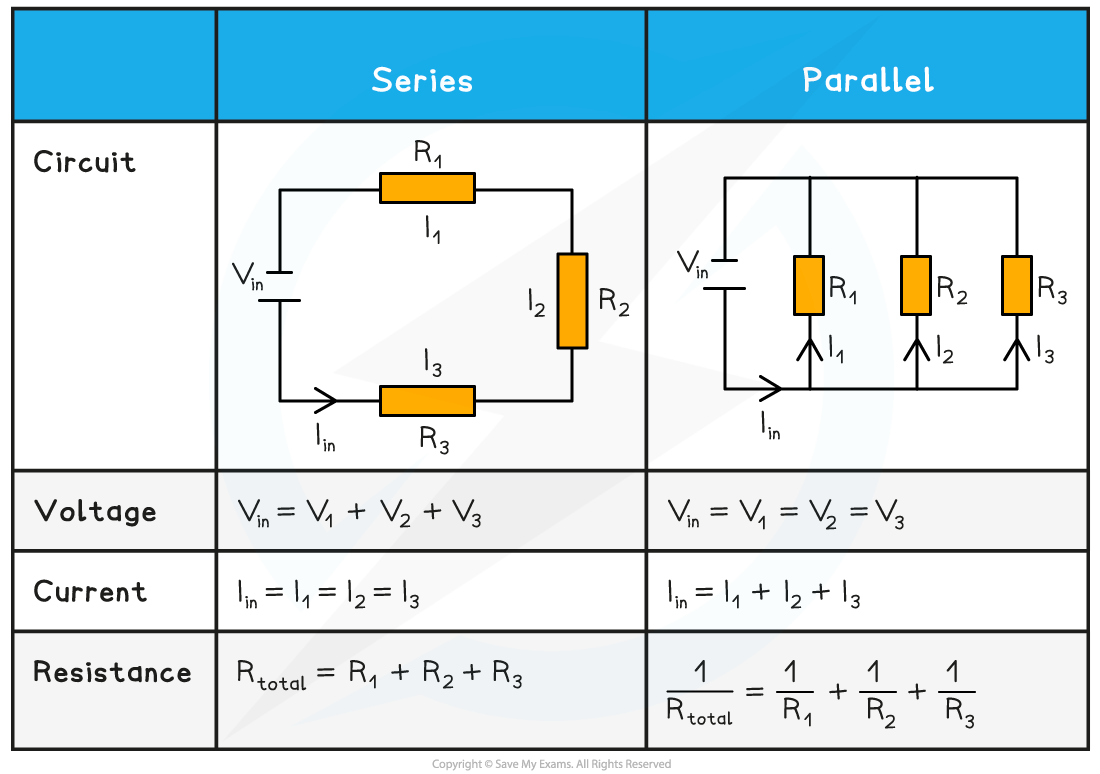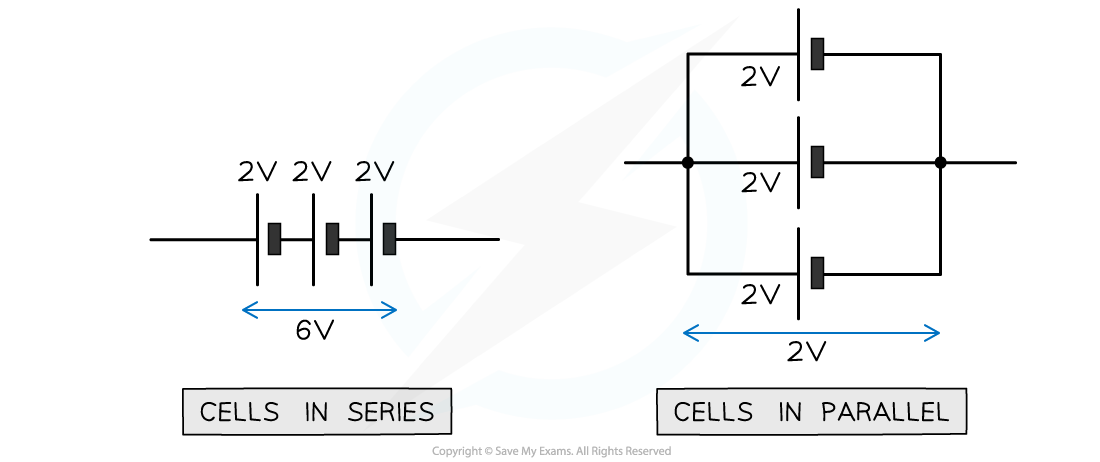# IB DP Physics: HL复习笔记5.2.7 Series & Parallel Circuits

### Series & Parallel Circuits

• The following statements for series and parallel circuits are a consequence of Kirchhoff's first and second law
• In a series circuit:
• The current is the same at any point
• The potential difference is split across all components depending on their resistance
• In a parallel circuit:
• The total current is equal to the sum of the currents in each parallel branch of the circuit
• The potential difference across each loop is the same

#### Series and Parallel Circuit Rules

• The table below summarises the rules for calculating current, potential difference (i.e. voltage) and resistance within series and parallel circuitsAdvantages of Parallel Circuits

• Parallel circuits are incredibly useful for home wiring systems
• A single power source supplies all lights and appliances with the same potential difference
• If one light breaks, current can still flow through the rest of the lights and appliances

#### Series and Parallel Cells

• Cells can also be connected in series or parallel
• If the cells are connected in series, the total potential difference between the ends of the chain of cells is the sum of the potential difference across each cell
• If the cells are connected in parallel, the total potential difference across the arrangement is the same as for one cell